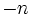# Tour:Group of integers

PREVIOUS: Isomorphic groups| UP: Introduction four (beginners)| NEXT: Group of integers modulo n
General instructions for the tour | Pedagogical notes for the tour | Pedagogical notes for this part
WHAT YOU NEED TO DO: Read and understand the definition of the group of integers.

## Definition

### Usual definition

The group of integers, typically denoted$\mathbb{Z}$, is defined as follows:

• The underlying set is the set of all integers
• The group operation is integer addition
• The identity element is the integer$0$
• The inverse map is the additive inverse, sending an integer$n$ to the integer$-n$

In the 4-tuple notation, the group of integers in the group$(\mathbb{Z},+, 0, -)$.